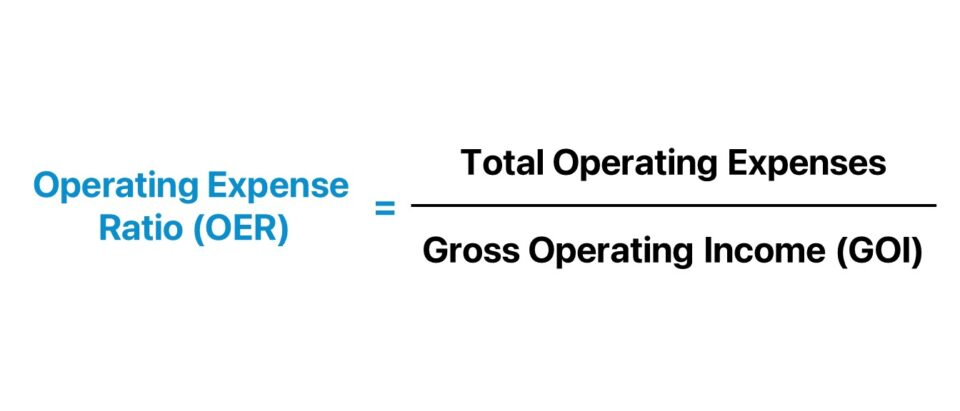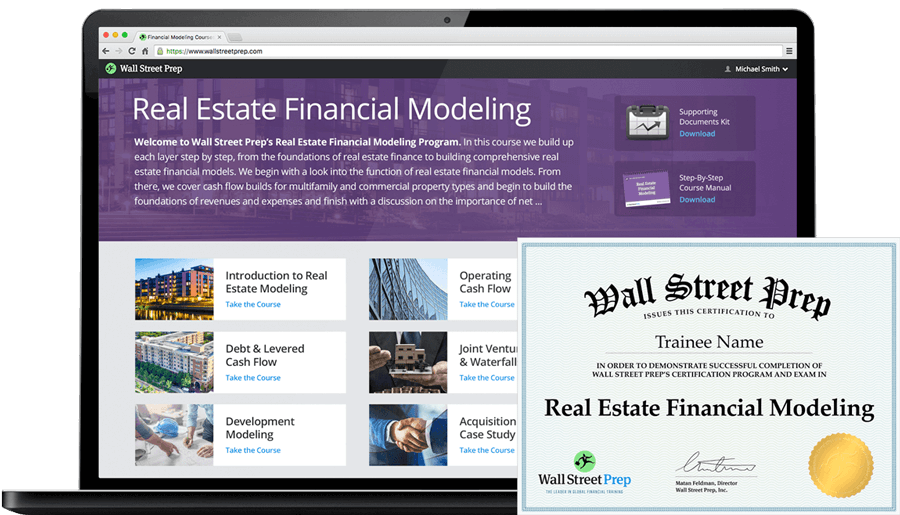Welcome to Wall Street Prep! Use code at checkout for 15% off.# Operating Expense Ratio (OER)

Guide to Understanding Operating Expense Ratio (OER)## How to Calculate Operating Expense Ratio?

The operating expense ratio (OER) is determined by dividing a real estate property’s operating expenses by its gross operating income (GOI).

• Low Operating Expense Ratio → A lower OER indicates the property is run efficiently by management (and vice versa for a higher OER ratio).
• High Operating Expense Ratio → If a property’s OER is higher, a substantial percentage of the property’s income is reduced by its ongoing operating expenses. In effect, the margins on the property investment decline, which causes the returns to the real estate investor to decrease — all else being equal.

The operating expense ratio (OER) can be calculated using the following four-step process.

1. Determine the Operating Expenses → The operating expenses of a property include maintenance, repairs, property management fees, utilities, insurance, property taxes, and other costs incurred while running the property.
2. Calculate Gross Operating Income (GOI) → The gross operating income (GOI) is the total income that a property generates before expenses. GOI is usually composed primarily of rent payments collected from tenants, but any other sources of income must also be included, e.g. application fees, amenities fees, laundry fees, parking permits, or other on-premise services.
3. Divide the Operating Expenses by the Gross Operating Income (GOI) → The output is the operating expense ratio (OER). However, the resulting figure will be in decimal format.
4. Convert into Percentage → By multiplying the prior step by 100, the OER is converted into a percentage.## Operating Expense Ratio Formula (OER)

The operating expense ratio (OER) formula is as follows.

Operating Expense Ratio (OER) = Total Operating Expenses ÷ Gross Operating Income (GOI)

Since the OER formula compares an investment property’s operating expenses to its gross operating income (GOI), the ratio shows the percentage of a property’s gross operating income that can “cover” its operating expense burden.

## What is a Good Operating Expense Ratio?

Conceptually, the operating expense ratio (OER) illustrates the percentage of a property’s gross income that must be spent towards paying off operating expenses.

Therefore, a lower operating expense ratio (OER) is preferred because that implies a greater percentage of the property’s gross income remains as profit upon deducting operating expenses.

Since the operating expense ratio (OER) focuses on the operational efficiency of the property, neither financing costs such as mortgage payments and interest nor capital expenditures (Capex) are part of the calculation.

One caveat to a low OER is that the ratio can be reduced by limited reinvestment activity, even for required maintenance Capex, which could potentially cause other issues.

## Operating Expense Ratio vs. Cap Rate: What is the Difference?

The operating expense ratio (OER) and the capitalization rate, or “cap rate”, are two real estate metrics that serve distinct purposes and offer different perspectives on a potential property investment.

1. Operating Expense Ratio (OER) → The OER is a measure of operational efficiency and computes the proportion of a property’s gross operating income (GOI) that is spent on operating expenses.
2. Cap Rate → In contrast, the cap rate is a measure of a property’s potential return on investment. To calculate the cap rate, the property’s net operating income (NOI) is divided by the property’s current market value, resulting in the implied yield.

Therefore, the use case of OER is to better grasp the cost efficiency of a property’s operations, whereas the cap rate is a method used to estimate the potential return on a property investment.

## Operating Expense Ratio Calculator

We’ll now move to a modeling exercise, which you can access by filling out the form below.#### Get the Excel Template!Submitting...

## Operating Expense Ratio Calculation Example (OER)

Suppose a real estate investment firm acquired a residential building with a total of 100 units and a market rate rent of \$4k per month.

• Total Number of Units = 100 Units
• Monthly Rent = \$4,000

The product of the total number of units and the monthly rent equals \$400k, which is the building’s total rental income per month.

Since we’re calculating the operating expense ratio (OER) on an annual basis, we’ll convert the monthly rental income into an annualized figure by multiplying by 12, i.e. each lease is a twelve-month arrangement.

• Total Rental Income – Monthly = 100 × \$4,000 = \$400,000
• Total Rental Income – Annual = \$400,000 ×12 = \$4.8 million

Furthermore, we’ll assume the other income earned on the side by the property amounts to \$200k, which we’ll simply add to our total income.

Upon computing the sum, the potential gross income (PGI)  comes out to \$5 million.

• Potential Gross Income (PGI) = \$4.8 million + \$200k = \$5 million

However, the potential gross income (PGI) metric assumes there are no vacancy losses or credit losses (i.e. collection issues), which is an inevitable part of property management, regardless of the methods used to mitigate risk.

For the vacancy and credit losses, we’ll attach an 8.0% assumption (of PGI) to estimate the projected losses.

• Vacancy and Credit Losses = 8.0% × \$5 million = (\$400k)

The gross operating income (GOI) is \$4.6 million, which we determined by adjusting the potential gross income (PGI) by the vacancy and credit losses.

• Gross Operating Income (GOI) = \$5 million – \$400k = \$4.6 million

Our model is now missing only one assumption – the total operating expenses – which we’ll assume to be \$8 million.

• Total Operating Expenses = \$8 million

In conclusion, we’ll divide the residential building’s gross operating income (GOI) by the total operating expenses to arrive at an operating expense ratio (OER) of 57.5%.

• Operating Expense Ratio (OER) = \$4.6 million ÷ \$8 million = 57.5%20+ Hours of Online Video Training

### Master Real Estate Financial Modeling

This program breaks down everything you need to build and interpret real estate finance models. Used at the world's leading real estate private equity firms and academic institutions.

Inline Feedbacks